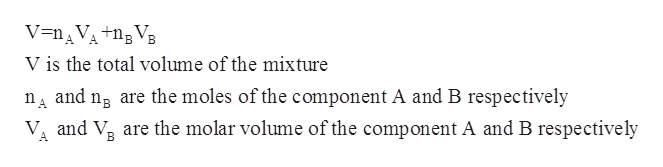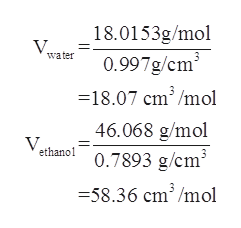# The partial molar volumes of water and ethanol in a solution with xH2O=0.45 at 25 ∘C are 17.0 and 57.0 cm3⋅mol−1, respectively. Calculate the volume change upon mixing sufficient ethanol with 4.30 mol of water to give this concentration. The densities of water and ethanol are 0.997 and 0.7893 g⋅cm−3, respectively, at this temperature.Express your answer to two significant figures and include the appropriate units.

Question
230 views
The partial molar volumes of water and ethanol in a solution with xH2O=0.45 at 25 ∘C are 17.0 and 57.0 cm3⋅mol−1, respectively.
Calculate the volume change upon mixing sufficient ethanol with 4.30 mol of water to give this concentration. The densities of water and ethanol are 0.997 and 0.7893 g⋅cm−3, respectively, at this temperature.
Express your answer to two significant figures and include the appropriate units.
check_circle

Step 1

In a mixture containing two miscible liquids, the partial molar volume refers to the contribution which is made by a given component that is present in the mixture to the overall volume of the given solution.

Step 2

In a two- component system which is maintained at a constant temperature, the volume of the mixture can be calculated using the following formula as follows:help_outlineImage TranscriptioncloseV=nAVA +nBVB V is the total volume of the mixture nA and ng are the moles of the component A and B respectively VA and V are the molar volume of the component A and B respectively fullscreen
Step 3

The molar volume of ethanol and water...help_outlineImage Transcriptionclose18.0153g/mol 0.997g/cm - 18.07 cm3/mol water 46.068 g/mol 0.7893 g/cm3 V ethano -58.36 cm3/mol fullscreen

### Want to see the full answer?

See Solution

#### Want to see this answer and more?

Solutions are written by subject experts who are available 24/7. Questions are typically answered within 1 hour.*

See Solution
*Response times may vary by subject and question.
Tagged in

### Physical Chemistry